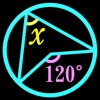# Find Angles! - Math questions of Plane Geometry - By Gakko Net Inc. - Lumos Educational App Store51
Price -Free
\$0

#### DESCRIPTION:

Find Angles! Once you have completed the basic questions from Stage 1 to Stage 4, try to solve the challenging questions on the Final Stage! If you can't solve a question, you can check the hint of the question or ask the answer to your friends via Twitter. 'Angles' contains following contents: --- -The sum of the Angles of a triangle -Exterior angle of a triangle -Isosceles triangle -Right-angled triangle -Vertical angle -Corresponding angle -Alternate angle -Parallelogram -The sum of the Angles of a quadrilateral -The sum of the Angles of a polygon -Inscribed angles -

#### OVERVIEW:

Find Angles! - Math questions of Plane Geometry - By Gakko Net Inc. is a free educational mobile app By Gakko Net Inc..It helps students in grades 7 practice the following standards 7.G.5.

This page not only allows students and teachers download Find Angles! - Math questions of Plane Geometry - By Gakko Net Inc. but also find engaging Sample Questions, Videos, Pins, Worksheets, Books related to the following topics.

1. 7.G.5 : Use facts about supplementary, complementary, vertical, and adjacent angles in a multi-step problem to write and solve simple equations for an unknown angle in a figure. .

7

#### STANDARDS:

7.G.5

Developer: Gakko Net Inc.

Software Version:

Category: Education

### RELATED APPSEdSearch WebSearch